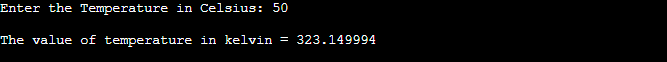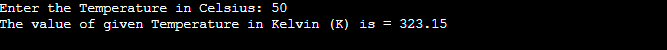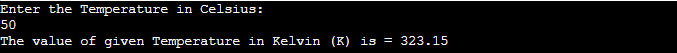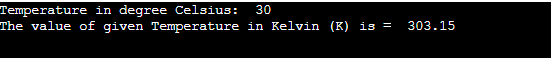Q:

# Program to convert temperature degree from Celsius to Kelvin

In this article, we will discuss the program to convert temperature degree from Celsius to Kelvin in different programming languages.

But before writing the programs, let's first discuss Celsius, Kelvin, and the formula to convert temperature degree from Celsius to Kelvin.

### What is Celsius?

Celsius is the unit to measure the temperature. It is also known as Centigrade. The symbol °C (read as degree Celsius or Centigrade) represents it. There is no difference between Centigrade and Celsius. Celsius was invented by the Andes Celsius. It is also called Centigrade because the scale is defined between 0 to 100 degree.

In the Celsius scale, 0°C represents the melting point of the ice, while 100°C represents the boiling point of water. The absolute zero in the Celsius scale is 273.15°C.

### What is Kelvin?

It is an SI base unit of temperature. Its unit symbol is K. Unlike Celsius, Kelvin is not written in degree.

To find the Kelvin temperature from Celsius, we can use the below formula -

K = 0C + 273

To convert the temperature from Celsius to Kelvin, the value is to be increased numerically by 273. Zero Kelvin (0K) is equal to -2730 C.

### Formula to convert temperature degree from Celsius to Kelvin

The formula of converting the temperature degree from Celsius to Kelvin is given as follows -

Celsius to Kelvin, K = C + 273.15

## Programs to convert temperature degree from Celsius to Kelvin

Now, let's see the programs to convert temperature degree from Celsius to Kelvin. The approach that we are using in the programs is given as follows -

• The temperature is given in the degree Celsius.
• After that, we will use the below formula to convert it into Kelvin -

K = C + 273.15

Program: Write a program to convert temperature degree from Celsius to Kelvin in C.

``````#include<stdio.h>
//function to convert temperature from Celsius into kelvin
float temp(float celsius)
{
float kelvin;
kelvin = celsius + 273.15; //formula to covert temperature from Celsius into kelvin
printf ("\nThe value of temperature in kelvin = %f", kelvin);
}
int main ()
{
float celsius;
printf ("Enter the Temperature in Celsius: ");
scanf ("%f", &celsius);
temp(celsius);
return (0);
}   ``````

### OutputProgram: Write a program to convert temperature degree from Celsius to Kelvin in C++.

``````#include <iostream>
using namespace std;
// function to convert temperature from degree Celsius to Kelvin
float temp(float celsius)
{
return (celsius + 273.15);
}
int main()
{
float celsius;
cout <<"Enter the Temperature in Celsius: ";
cin >>celsius;
cout << "The value of given Temperature in Kelvin (K) is = " << temp(celsius);

return 0;
}  ``````

### OutputProgram: Write a program to convert temperature degree from Celsius to Kelvin in C#.

``````using System;
class JTP {
// function to convert temperature from degree Celsius to Kelvin
static float temp(float celsius)
{
return (float)(celsius + 273.15);
}
public static void Main()
{
float celsius;
Console.WriteLine("Enter the Temperature in Celsius: ");
Console.WriteLine("The value of given Temperature in Kelvin (K) is = " + temp(celsius));
}
}  ``````

### OutputProgram: Write a program to convert temperature degree from Celsius to Kelvin in Java.

``````import java.util.*;
class Temp{
// function to convert temperature from degree Celsius to Kelvin
static float temperature(float celsius)
{
return (float)(celsius + 273.15);
}
public static void main (String[] args)
{
float celsius;
System.out.println("Enter the Temperature in Celsius: ");
Scanner sc = new Scanner(System.in);
celsius = sc.nextFloat();
System.out.println("The value of given Temperature in Kelvin (K) is = " + temperature(celsius));

}
}  ``````

### OutputProgram: Write a program to convert temperature degree from Celsius to Kelvin in JavaScript.

``````<html>
<body>
<script>
// function to convert temperature from degree Celsius to Kelvin
function temp(celsius)
{
return (celsius + 273.15);
}
var celsius = parseFloat(prompt("Enter the value of Temperature in Celsius: "));
document.write("The value of given Temperature in Kelvin (K) is = " + temp(celsius));
</script>

</body>
</html>  ``````

### Output

After the execution of the above code, the output will be -After entering the value and clicking OK, the output will be -Program: Write a program to convert temperature degree from Celsius to Kelvin in Python.

``````# Function to convert temperature from degree Celsius to Kelvin
def temp(celsius):
return (celsius + 273.15)
celsius = 30
print("Temperature in degree Celsius: ", celsius)
print("The value of given Temperature in Kelvin (K) is = " , temp(celsius))  ``````

### Output

After the execution of the above code, the output will be -Program: Write a program to convert temperature degree from Celsius to Kelvin in PHP.

``````<?php

// function to convert temperature from degree Celsius to Kelvin
function temp(\$celsius)
{
return (\$celsius + 273.15);
}
\$celsius = 30;
echo "Temperature in degree Celsius: " , \$celsius , "<br/>";
echo "The value of given Temperature in Kelvin (K) is = " , temp(\$celsius);
?>  ``````

### Output

After the execution of the above code, the output will be -